### Python Lab Tutorials: Getting Started With Python

This section covers the syntax and functions in Python that are needed to solve the majority of the labs. These labs will use Python 3

Google Colab is a free Jupyter Notebook to run Python code online without any installations. Alternatively, Python can be downloaded directly to your computer using anaconda.com or python.org

To get started, go to colab.research.google.com and sign in with a Google account, then press New Notebook to open a new notebook. All newly created notebooks are automatically stored in a new folder in My Drive called Colab Notebooks

1. Colab supports two types of blocks: text blocks and code blocks. To create a new block, either click the Code or Text buttons at the top left or hover the cursor over the top or bottom of a block and click the buttons
2. Text Block – double click or press enter to edit the text block. Text blocks can be used to write text or to insert images and hyperlinks
3. Code Block – runs Python code. To run the code, press the play button on the left or use one of the following shortcuts:
1. CTRL + ENTER – run the selected code cell
2. SHIFT + ENTER – run the selected code cell and go to the next cell
4. Runtime Settings – the Runtime menu in the top bar provides options to run the code:
1. Restart runtime – restarts the notebook and clears all variables
2. Factory Reset Runtime – restarts the notebook and clears all variables and all imported files
3. Run all – run all cells sequentially

While Colab has many pre-installed libraries, any additional libraries must be installed using `!pip install <library name>`

Some features may only available in newer versions of a library and so upgrading a library may be necessary. Run `!pip install <library name> --upgrade` and then Restart Runtime

``````!pip install sympy                 # To install Sympy

### Python Basics

Python is a high-level programming language that emphasizes code readability. It is case sensitive (ie. uppercase and lowercase letters are not interchangeable) and indent sensitive (ie. the number of indents and spaces are important). Semicolons are not needed in Python but can be used. Python is 0-indexed, which means the first element of a list is at index 0

Comments are lines of code preceded by a hashtag (#), which are not executed and is used to provide explanatory information

``# This is a comment``

#### Print

`print()` is used to display output to the user. Multiple values can be printed on the same line by separating it by commas

``````print("Hello World")    # Hello World
print("Hello","World")  # Hello World
print(100)              # 100
print(1+1)              # 2
print(1,2,3)            # 1 2 3``````

#### Basic Math Operations

Let a, b, and n represent numeric values

• Addition: `a+b`
• Subtraction: `a-b`
• Multiplication: `a*b`
• Division: `a/b`
• Integer Division: `a//b`
• Remainder (mod): `a%b`
• Exponent: `a**b`
• Round to Integer: `round(a)`
• Round to `n` Decimal Places: `round(a,n)`
• Absolute Value: `abs(a)`
• Sum of List: `sum([a,b,...])`
• Min of List: `min([a,b,...])`
• Max of List: `max([a,b,...])`
``````print("Addition:",5 + 3)              # 8
print("Subtraction:",5 - 3)           # 2
print("Multiplication:",5 * 3)        # 15
print("Division:",5 / 3)              # 1.66...
print("Integer Division:",5 // 3)     # 1
print("Remainder:",5 % 3)             # 2
print("Exponent:",5 ** 3)             # 125
print("Round:",round(3.1415))         # 3
print("Round:",round(3.1415,2))       # 3.14
print("Absolute Value:",abs(-10))     # 10
print("Sum:",sum([1,2,3,4]))          # 10
print("Min:",min([1,2,3,4]))          # 1
print("Max:",max([1,2,3,4]))          # 4``````

#### Variables

Variables are names that store values. The values can be changed and accessed by calling their name. Variables can be assigned and changed as follows: `name = value`

``````myString = "Old String"
myString = 'New String'
print(myString) # New String

a = 2
b = 3
c = a*b
print(c)   # 6``````

Note that strings in Python can be created using either single quotes (`'`) or double quotes (`"`), that is, `"this string"` is equal to `'this string'`

#### Lists

A list is a sequence of values stored in order. Values in lists can be indexed, that is it can be accessed by its index or its position in the list. The first element in a list is at index 0

Let a,b represent numerical values

• Creating Empty List: `myList = []`
• Creating Non-Empty List: `myList = [a,b,...]`
• Length of List: `len(myList)`
• Add Element to End of List: `myList.append(a)`
• Access Element at Index a: `myList[a]`
• Matrix: `matrix = [[a,...],[b,...]]`
• Access Element at Index (a,b): ` matrix[a][b]`
``````myList = []
print("Empty:",myList)                 # []
myList = [1,2,3]
print("Non-Empty:",myList)             # [1,2,3]
print("Length:",len(myList))           # 3
myList.append(4)
print("Append:",myList)                # [1,2,3,4]
print("myList:",myList)          # 1
myList = 9
print("Change Index 0:",myList)        # [9,2,3,4]

matrix = [[1,2,3],[4,5,6],[7,8,9]]
print("matrix:",matrix)                # [[1,2,3],[4,5,6],[7,8,9]]
print("matrix:",matrix)    # 5
matrix = 9
print("Change Index (1,1):",matrix)    # [[1,2,3],[4,9,6],[7,8,9]]``````

Python also has a tuple, which is similar to a list except its elements are final and can not be changed. Tuples are enclosed by round brackets, whereas lists are enclosed by square brackets. `myTuple = ()`

## If – Else Statements

A condition is an expression that evaluates to either True or False and a conditional statement will run a block of code only if the condition evaluates to True

The `if` keyword is the syntax for a conditional statement. There can only be one `if `keyword for each if-else statement. Note that all indented code immediately after the if condition will run if the condition evaluates to True

``````if <condition>:
<code to run if condition is True>``````

The `else` keyword is optional and does not have a condition, therefore it will only run if all the `if` and `elif` statements are False. There can only be one `else` keyword per if-else statement and it must be after the `if `keyword

``````if <condition>:
<code to run if condition is True>
else:
<code to run if condition is False>``````

The `elif` keyword is optional and is similar to the `if` keyword. It allows for more conditional statements. There can be many `elif` statements, all of which must be declared after the `if` keyword and before the `else` keyword

``````if <condition1>:
<code to run if condition1 is True>
elif <condition2>:
<code to run if condition2 is True>
else:
<code to run if all conditions are False>``````

For example

``````a = 2; b = 3;
if a != 0:
print("a is not equal to 0")
elif a == b:
print("a is equal to b")
elif a > b:
print("a is greater than b")
elif a <= -10:
print("a is less than or equal to -10")
else:
print("all if and elif are False")``````

## Loops

`for` loops are used to repeat a block of code for a known number of times. `while` loops are used when the number of iterations is unknown and will loop until the condition is met. An iteration is one full execution of the code within a loop

To exit a loop, use the `break` keyword

### For Loops

``````for <iteration variable> in <range or list>:
<code to loop>``````

#### Range

Use `range` to loop an iterating variable through a range, where the iterating variable will increment by 1 after each iteration

`for i in range(a):` will iterate `i` through the range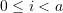``````for i in range(5):
print(i)``````

`for i in range(a,b):` will iterate `i` through the range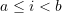``````for i in range(5,10):
print(i)``````

`for i in range(a,b,step_size):` changes the step size

``````for i in range(0,10,2):
print(i)``````

For example, create a list of the y-values of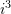from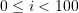:

``````myList= []
for i in range(0,100):
myList.append(i**3)
print(myList)  # [0, 1, 8, 27, 64,...]``````

#### Iterate Through A List

`for i in <list>:` will iterate through each element of a list, where `i` is the element of the list

For example, the following code replaces each element in `myList` with its twice

``````myList= [1,2,3,4,5]
k = 0
for i in myList:
myList[k] = i*2
k += 1
print(myList)  # [2, 4, 6, 8, 10]``````

### While Loops

``````while <condition>:
<code to run if while condition is True>``````

For example, create a list of the y-values offrom:

``````myList= []
i = 0
while i < 100:
myList.append(i**3)
i += 1
print(myList)  # [0, 1, 8, 27, 64,...]``````

While loops can run indefinitely if the condition is equal to True: `while True`

### List Comprehension

List Comprehensions are used to create a new list in one line

``<variable> = [<element of new list> for i in <range or list>]``

For example, create a list of the y-values offrom:

``````myList= [i**3 for i in range(0,100)]
print(myList)  # [0, 1, 8, 27, 64,...]``````

### Nested Loops

A nested loop is a loop within a loop, which is often used to iterate through a matrix. For each iteration of the outer loop, the inner loop executes to completion before moving to the next iteration of the outer loop

``````for <outer> in <range or list>:
for <inner> in <range or list>:
<code>``````

For example, print out the elements of the matrix:

``````matrix = [[1,2,3],[4,5,6],[7,8,9]]

# Option 1
for row in matrix:
for col in row:
print(col, end=" ")
print()  # New line after each row

# Option 2
for row in range(0,len(matrix)):
for col in range(0,len(matrix)):
print(matrix[row][col], end=" ")
print()  # New line after each row``````

`len(matrix)` returns the number of nested loops or the number of rows. `len(matrix)` returns the length of the first nested loop or the number of columns.

### User-Defined Functions

User defined functions start with the `def` keyword and are used to bundle code which can then be called repeatedly in the program. Functions can take in inputs and return outputs using the `return` keyword; it is also legal to have a function with no inputs and/or no outputs. All indented lines after `def` are in the function

``````def <name>(<inputs>):
<code>
return <output>``````

To call the function:

``````# No ouput returned
<name>(<inputs>)
# Ouput is returned
output = <name>(<inputs>)``````

For example, create a function for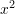named `f` that takes an input `x` and returns the value ofat `x`

``````def f(x):
return x**2
y = f(3)
print("f(3):",y)  # 9``````

Functions can take numerical values and lists as inputs. For example, create a function that takes a matrix as input and returns the sum of the diagonal components of the matrix

``````def diagonal_sum(M):
return sum(M[i][i] for i in range(len(M)))
A = [[1, 1], [2, 1]]
B = [[1, 2, 3], [4, 5, 6], [7, 8, 9]]
print(diagonal_sum(A))  # 2
print(diagonal_sum(B))  # 15``````

For example, the following function returns the sum of the first `i` terms in the list `x`

``````def i_sum(x,i):
return sum([x[n] for n in range(i)])
x = [1,2,3,4,5,6,7,8,9,10]
print(i_sum(x,3))  # 6``````